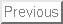## Divertor Fluid ModelsM. Day,B. Merriman,F. Najmabadi,R. W. Conn•Work performed under the auspices of the US DOE, contract W-7405-ENG-48
•LLNL, L-561, P.O. Box 808, Livermore, CA 94550
•Fusion Energy Research Programs, UC San Diego, La Jolla, CA 92093
• Abstract

The classical plasma electron heat flux can greatly overestimate the physical heat flux when the electron mean-free-path becomes long compared to the electron temperature gradient scale length. For fluid modelling of plasmas, a common remedy is to apply an artificial ``flux limiter'' that keeps the heat flux at a physically reasonable value in this long mean-free-path regime. The limiter is not derived from first principles and it introduces a poorly understood free parameter into the model. We study the effect of this parameter in our divertor plasma models to understand how it influences the computed solution. We investigate regimes of both short and long mean-free-path and consistently find large parameter sensitivity. Thus, without additional experimental or theoretical guidance in the choice of flux-limit parameter, flux limiting does not appear to provide an acceptable basis for predictive plasma fluid modelling.

1. Background

Early experiments with laser-heated plasmas demonstrated that the classical expressions for thermal conductivities lead to significantly overestimated electron heat fluxes in regimes of long mean-free-path. This observation led to the flux-limit models which are employed to study and predict divertor behavior. Typically, flux limiters impose a maximum allowable heat flux of the form,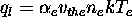(where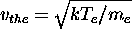is the electron thermal velocity, and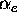is an arbitrary constant such that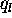remains less than the flux of thermal energy that could be carried by the electrons moving at their thermal speed). The limited heat flux is written as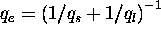, which is an arbitrary interpolation between the free-streaming and Spitzer values (here,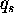is the classical Spitzer heat flux). The flux-limit parameter,, is adjusted according to some application-specific kinetic modelling or experimental data. For tokamak edge plasma modelling, values forrange typically from .12 to .50. In addition to flux-limiting models, there has been considerable theoretical effort to develop nonlocal heat conduction models. Reference 3 includes a critique of a large class of such models; we focus here only on the local flux-limited models widely used in divertor modelling.

We have developed a 1D fluid model[4,6] which uses the above flux-limiter expression in simulating experimental data taken from a variety of plasma conditions in the PISCES-A facility at UC San Diego. Using this model, we perform sensitivity analyses to characterize how the flux-limit parameter influences the computed solutions.

2. PISCES-A Model and Results

The PISCES-A facility has been described in detail elsewhere. Briefly, PISCES-A is a linear device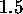m in length and 10 cm in diameter with plasma confined radially by a solenoidal magnetic field. Ions are not heated and remain near 2 eV (their Franck-Condon energy). The plasma regime for the electrons is in the range characteristic of divertor plasmas. In the gas-target experiments,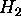is puffed into the system near the target, causing the plasma density to detach fully from the target and the electron temperature to collapse. Plasma detachment reduces the target heat load by more than 97%, and thus may be an attractive regime for divertor operation.

The model of Reference 4 and 6 describing these gas-target experiments employs classical fluid plasma transport parallel to the magnetic field for plasma density, plasma momentum and electron temperature, except that the electron heat flux is limited as described above, with flux-limit parameter,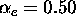. Radial transport effects are simulated by assuming radial scale lengths and diffusivities. Neutral H andtransport are modeled by diffusion. For the high-pressure ``gas-target'' regime (induced by strong externalpuffing), we performed sensitivity studies for. The resulting profiles are shown in Figure 1 forthrough the range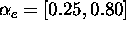. In these results, the target is toward the left and the inlet is toward the right of the 100 cm model domain.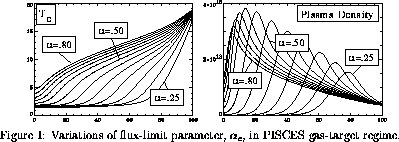We also simulated a low-pressure PISCES-A case (no externalpuffing). Our model successfully reproduces measured data for this case only after decreasingto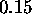. The profiles obtained by varyingthrough the range,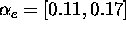, are shown in Figure 2.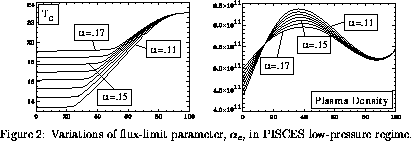In Figure 3 we plot the ratio, R, of the mean-free-path for Coulomb collisions to electron temperature gradient scale length for the high- and low-pressure PISCES-A experimental data (based on the computed solutions that best represent the experimental data). Where R exceeds unity the classical heat flux significantly overestimates the physical heat flux, and the flux limiter must significantly impact the fluid model.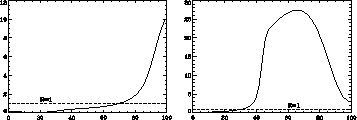Figure 3: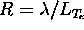for gas-target (left) and low-pressure (right) PISCES-A models.

In the high-pressure experiment, the mean-free-path is smaller than the temperature scale length (R<1) over most of the domain, except near the inlet. Even so, Figure 1 shows that variations in the flux limit parameter have remarkable effects on the solution profiles. In the low-pressure case, R>1 over a large part of the domain, and the sensitivity of the solution profiles tois even more pronounced. In the latter case, there is a strong relation betweenand the resulting predicted target temperature. It is clear from the both figures that small changes in, will ``tune'' the computed results. In applications of a similar model to a high power gas-target tokamak divertor regime, we further found that the relation between variations inand the resulting plasma profiles was not easily predictable. This makes it difficult to provide generally useful guidelines about the effect of the flux limit on the computed solutions.

3. Simple Analytic Model

A simple model for electron heat transport can be constructed to illustrate some basic effects of the limiter. We start from parallel transport equations for plasma density, momentum and energy. Assuming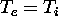and neglecting sources we can satisfy the steady state continuity and momentum equations with zero flow velocity and a constant plasma pressure. The steady heat transport equation then reduces to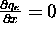. This equation can be integrated twice to obtain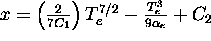, where we have absorbed all the physical constants into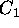,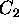and(andmay be set with Dirichlet boundary conditions). In Figure 4,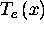is plotted for a range ofwith arbitrary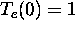and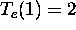. Note that there exists a critical, below which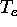cannot simultaneously satisfy the boundary values and\ remain single valued in x (so as to admit a physically relevant solution). In fact, for any ``flux-limiting'' model, if the conductivity,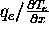, is strictly an increasing function ofand if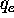remains bounded as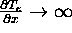, then there may be no solution which satisfies both boundary conditions for an arbitrarily large heat flux. EPS/toyFl.epsFlux limit variations in simple analytic model.toyFl.4 Note that the limited heat flux becomes an increasingly weaker function of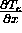as the local temperature gradient increases; this in turn requires much steeper gradients to carry the heat flux as(and thus the conductivity) decreases. In contrast, kinetic effects in the physical system tend to relax local gradients because hot particles tend to carry heat further into cool regions.

In the results for PISCES-A (Figures 1 and 2), decreasingleads to more steep temperature profiles, as expected from the simple model. Additionally, our models exhibit a threshold, beyond which steady solutions no longer exist, also as predicted by the simple arguments above. In the low-pressure regime, we could find no steady solutions for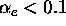.

4. Conclusions

We have shown that details of the particular flux-limiter implementation have significant impact on computed divertor plasma solutions in 1D. We analyzed a simple model for the limiter and found that it may not admit physically realistic solutions. Profiles computed with these flux-limited models can be ``tuned'' strongly via minor variations in the arbitrary parameter of the model. Based on this behavior, we conclude that flux-limiting does not provide an acceptable alternative to more detailed (kinetic) modelling for predictive plasma applications. Use of flux limiters should be accompanied by sensitivity studies such as those presented here, and may require additional experimental or theoretical input to select a proper value of the flux-limit parameters for predictive modelling, even when modelling within a restricted regime of plasma parameters.

References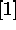R. H. Cohen, et al., Contrib. Plasma Phys. 34 (2/3), 198 (1994)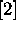J. F. Luciani, et al., Phys. Rev. Lett. 51, 1664 (1983)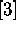M. K. Prasad and D. S. Kershaw, Phys. Fluids B 1 (12), 2430 (1989)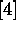M. Day, Ph.D. Thesis, UCLA (1995)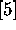D. M. Goebel, et al., J. Nucl. Mat. 121, 27 (1984)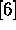L. Schmitz, et al., Physics of Plasmas 8, 3081 (1995)